# NCERT Solutions For Class 10 Maths Chapter 13 Exercise 13.5

Go back to  'Surface Areas and Volumes'

## Chapter 13 Ex.13.5 Question 1

A copper wire, $$3\,\rm{mm}$$ in diameter, is wound about a cylinder whose length is $$12\,\rm{cm}$$, and diameter $$10\,\rm{cm}$$, so as to cover the curved surface of the cylinder. Find the length and mass of the wire, assuming the density of copper to be $$8.88\,\rm{g\,per}$$ $$\rm{cm^3}$$

### Solution

What is Known?

Diameter of the copper wire is $$3\rm{mm.}$$ Length of the cylinder is $$12\rm{cm}$$ and diameter is $$10\rm{cm.}$$ Density of copper is $$8.88 \,\,\text{g per} \,\rm{cm^3}$$

What is Unknown?

Length and mass of the wire

Reasoning:

Draw a figure to visualize the cylinder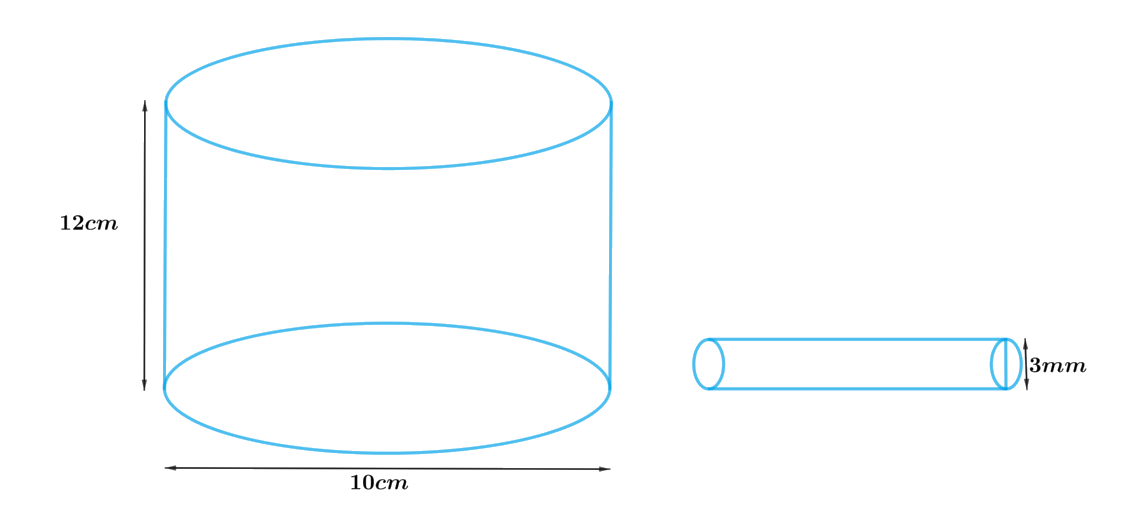To find length of the wire:

Since the curved surface of the cylinder to be covered with the copper wire then one round of copper wire will cover same height of cylinder as of the width (diameter) of the wire.

Therefore, Length of the wire $$\times$$ diameter the of wire $$=$$ CSA of the cylinder

We will find the CSA of the cylinder by using formula;

CSA of the cylinder $$= 2\pi rh$$

where $$r$$ and $$h$$ are radius and height of the cylinder respectively.

To find mass of the wire:

Mass $$=$$ Density $$\times$$ Volume

As the density of the copper is given, we need to find volume of the wire first.

Since the wire is cylindrical in shape

Therefore, Volume of the wire $$=$$ volume of the cylinder

We will find the volume of the cylindrical wire by using formula;

Volume of the cylinder $$= \pi {r_1}^2{h_1}$$

where $$r1$$ and $$h1$$ are the radius and the length of the cylindrical wire

Steps:

Height of cylinder,$$h = 12cm$$

Diameter of cylinder,$$d = 10cm$$

Radius of cylinder,\begin{align}r = \frac{{10cm}}{2} = 5cm\end{align}

Diameter (width) of wire\begin{align}{d_1} = 3mm = \frac{3}{{10}}cm = 0.3cm\end{align}

Radius of wire\begin{align}{r_1} = \frac{{0.3cm}}{2} = 0.15cm\end{align}

Let the length of the wire be $$h1$$

Since curved surface of the cylinder to be covered with the wire

Length of the wire $$\times$$ diameter the of wire $$=$$ CSA of the cylinder

\begin{align}{d_1} \times {h_1} &= 2\pi rh\\{h_1} &= \frac{{2\pi rh}}{{{d_1}}}\\&= \frac{{2 \times 3.14 \times 5cm \times 12cm}}{{0.3cm}}\\&= 1256cm\end{align}

Volume of the wire $$=$$ volume of the cylinder

\begin{align} & =\pi {{r}_{1}}^{2}{{h}_{1}} \\ & =\left[ \begin{array} & 3.14\times 0.15cm\times \\ 0.15cm\times 1256cm \\ \end{array} \right] \\ & =88.7364c{{m}^{3}} \\\end{align}

Density of copper$$= 8.8g/c{m^3}$$

Mass of the copper Wire $$=$$ Density $$\times$$ volume

\begin{align}&= 8.88g/c{m^3} \times 88.7364c{m^3}\\&= 787.97923g\end{align}

Length of the wire is $$1256cm$$ and Mass is $$788g{\rm{ }}$$ (approx.)

## Chapter 13 Ex.13.5 Question 2

A right triangle, whose sides are $$3 \,\rm{cm}$$ and $$4\,\rm{cm}$$ (other than hypotenuse) is made to revolve about its hypotenuse. Find the volume and surface area of the double cone so formed. (Choose value of π as found appropriate.)

### Solution

What is Known?

A right triangle whose sides are $$3 \,\rm{cm}$$ and $$4\,\rm{cm}$$ (other than hypotenuse) is made to revolve about its hypotenuse to form cone.

What is Unknown?

The volume and surface area of the double cone formed.

Reasoning:

Draw a figure to visualize the double cone formed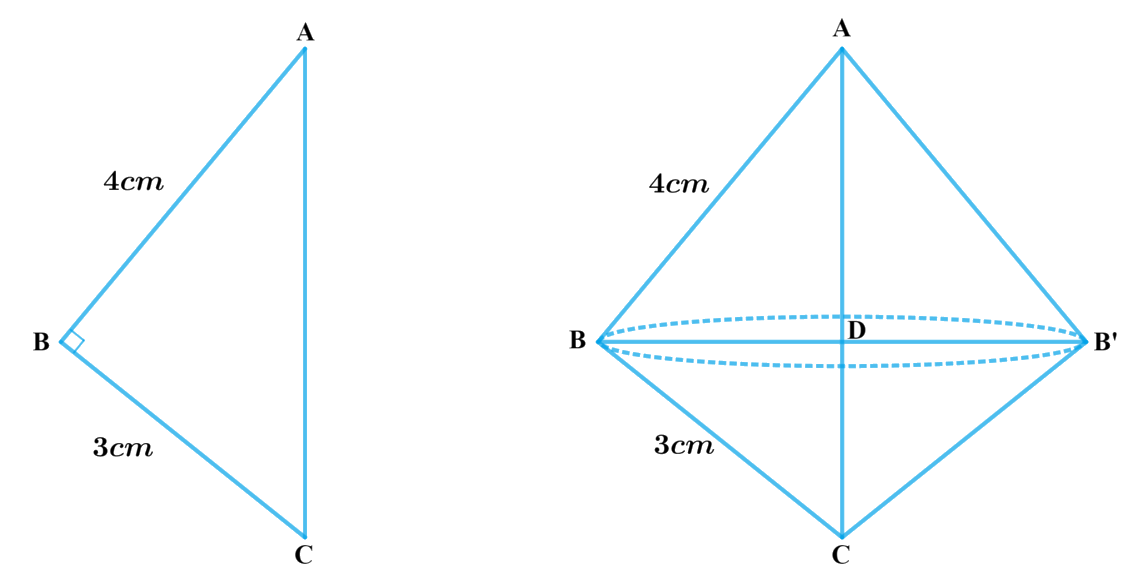In order to find the volume and surface area, we need to find $$BD$$ or radius of the double cone

From the figure it’s clear that $$BD \bot AC$$

To find $$BD$$

(i) We first find $$AC$$ using Pythagoras theorem

\begin{align}A{C^2} &= A{B^2} + B{C^2}\\AC &= \sqrt {A{B^2} + B{C^2}} \end{align}

(ii) Using AA criterion of similarity

Prove

\begin{align}&{\Delta ABC}\sim{\Delta BDC}\\&{ \frac{{AB}}{{BD}} = \frac{{AC}}{{BC}}}\end{align}

(Corresponding Sides of similar triangles are in proportion)

\begin{align}\text{Radius or}\, BD = \frac{{AB}}{{BC}} \times BC\end{align}

Since we know $$AB, AC$$ and $$BC; BD$$ can be found out

Since double cone is made by joining $$2$$ cones by their bases

Therefore Volume of double cone $$=$$ Volume of Cone $$ABB$$ $$+$$ Volume of Cone $$BCB$$

We will find the volume of the cone by using formulae;

Volume of the cone\begin{align} = \frac{1}{3}\pi {r^2}h\end{align}

where $$r$$ and $$h$$ are the radius and height of the cone respectively.

Visually from the figure it’s clear that CSA of double cone includes CSA of both the cones

Therefore, CSA of double Cone $$=$$ CSA of cone $$ABB’ +$$ CSA of cone $$BCB’$$

We will find the CSA of the cone by using formulae;

CSA of frustum of a cone$$= \pi rl$$

where $$r$$ and $$l$$ are the radius and slant height of the cone respectively.

Steps:

In $$\Delta ABC$$ right-angled at $$B$$

\begin{align}A{C^2} &= A{B^2} + B{C^2}\\AC &= \sqrt {{{\left( {3cm} \right)}^2} + {{\left( {4cm} \right)}^2}} \\&= \sqrt {9c{m^2} + 16c{m^2}} \\&= \sqrt {25c{m^2}} \\&= 5cm\end{align}

Consider $$\Delta ABC$$ and $$\Delta BDC$$

\begin{align}\angle ABC &= \angle CDB = 90^\circ {\rm{ }}\left( {BD \bot AC} \right)\\\angle BCA &= \angle BCD{\rm{}}\left( {{\rm{common}}} \right)\end{align}

By AA criterion of similarity $$\Delta ABC \sim \Delta BDC$$

Therefore,

\begin{align}\frac{{AB}}{{BD}} = \frac{{AC}}{{BC}}\end{align} (Corresponding sides of similar triangles are in proportion)

\begin{align}BD &= \frac{{AB \times BC}}{{AC}}\\&= \frac{{3cm \times 4cm}}{{5cm}}\\&= \frac{{12}}{5}cm\\&= 2.4cm\end{align}

Volume of double cone $$=$$ Volume of Cone ABB’ $$+$$ Volume of Cone BCB’

\begin{align}&= \frac{1}{3}\! \times \!\pi {(BD)^2} \times AD + \frac{1}{3}\pi {(BD)^2}\! \times\! DC\\&= \frac{1}{3} \times \pi {(BD)^2}\left[ {AD + DC} \right]\\&= \frac{1}{3} \times \pi {(BD)^2} \times AD\\&= \frac{1}{3} \times 3.14 \times 2.4cm \times 2.4cm \times 5cm\\&= \frac{{90.432}}{3}c{m^3}\\&= 30.144c{m^3}\\&= 30.14c{m^3}\end{align}

CSA of double Cone $$=$$ CSA of cone ABB’ $$+$$ CSA of cone BCB’

\begin{align}&= \pi\!\times\!BD \!\times\!AB\!+\!\pi\! \times\!BD\!\times\!BC\\&= \pi \times BD\left[ {AB + BC} \right]\\&= 3.14 \times 2.4cm \times \left[ {3cm + 4cm} \right]\\&= 3.14 \times 2.4cm \times 7cm\\&= 52.752c{m^2}\\&= 52.75c{m^2}\end{align}

## Chapter 13 Ex.13.5 Question 3

A cistern, internally measuring $$150\,\rm{cm}\, \times$$ $$120\,\rm{cm}$$ $$×$$ $$110\,\rm{cm,}$$ has $$129600$$ $$\rm cm^3$$of water in it. Porous bricks are placed in the water until the cistern is full to the brim. Each brick absorbs one-seventeenth of its own volume of water. How many bricks can be put in without overflowing the water, each brick being $$22.5\,\rm{cm}\,×$$ $$7.5\,\rm{cm}\, ×$$ $$6.5\,\rm{cm}$$ ?

### Solution

What is Known?

A cistern internally measuring $$150\,\rm{cm }\,\times$$  $$120\,\rm{cm }\, \times$$ $$110\,\rm{cm}$$ has $$129600$$ $$\rm{c{m^3}}$$ of water in it. Each brick is of dimensions $$22.5 \times 7.5 \times 6.5\rm{cm}$$ and can absorbs one-seventeenth of its own volume of water.

What is Unknown?

Number of bricks which can be put in the cistern without overflowing the water.

Reasoning: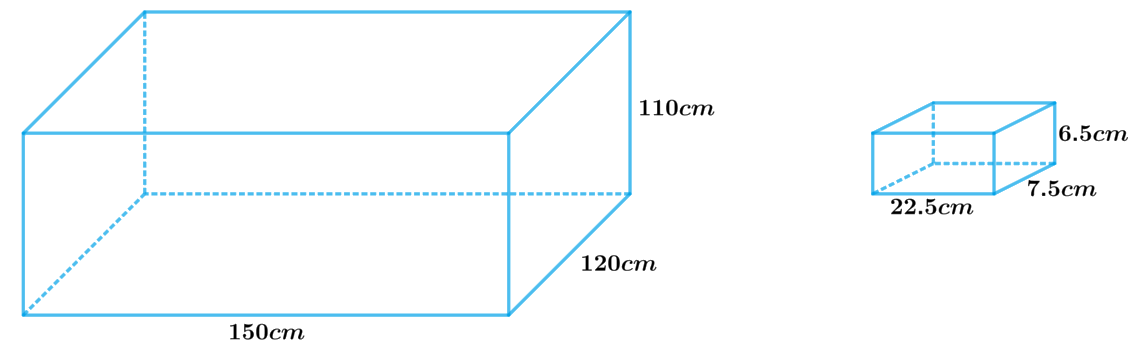Logically, the bricks have to occupy the space left in the cistern which is not occupied by water left in the cistern, after being absorbed by the bricks.

Therefore,

Internal volume of cistern $$=$$ (Volume of water in the cistern $$–$$ Volume of water absorbed by bricks) $$+$$ Volume of bricks

Internal volume of cistern $$=$$ Volume of water in cistern $$-$$ \begin{align}\frac{1}{{17}}\end{align} $$\times$$ Volume of  bricks $$+$$ volume of bricks

Hence,

Internal volume of cistern $$=$$ Volume of water in the cistern\begin{align} + \frac{{16}}{{17}}\end{align} volume of brick

Since the dimensions of the cistern and the brick are known which are cuboidal in shape

We will find the volume by using formula;

Volume of the cuboid $$= lbh$$

where $$l, b$$ and $$h$$ are length breadth and height of the cuboid respectively.

Steps:

Internal volume of the cistern \begin{align} = 150cm\! \times\! 120cm \!\times\! 110cm = 1980000c{m^3}\end{align}

Volume of water in the cistern $$= 129600c{m^3}$$

Volume of one brick

\begin{align}& = 22.5cm \times 7.5cm \times 6.5cm \\&= 1069.875c{m^3}\end{align}

Let the required number of bricks be $$‘x’$$

Volume of $$x$$ bricks \begin{align} = x \times 1069.875c{m^3}\end{align}

Internal volume of cistern $$=$$ Volume of water in the cistern $$+ \frac{{16}}{{17}}$$ volume of $$x$$ bricks

\begin{align}1980000c{m^3} &= 129600c{m^3} + \frac{{16}}{{17}} \times x \times 1096.875c{m^3}\\ x &= \frac{{\left( {1980000c{m^3} - 129600c{m^3}} \right) \times 17}}{{16 \times 1096.875c{m^3}}}\\ &= \frac{{1850400c{m^3} \times 17}}{{16 \times 1096.875c{m^3}}}\\ &= 1792.41\end{align}

Since the number of bricks should be a whole number, whole part of $$x$$ should be considered as the answer. Rounding off $$x$$ to the next whole number should not be considered as it will lead to overflowing of tank, if that extra fractional part is added to the cistern.

Therefore, number of bricks which can be put in the cistern without overflowing the water is $$1792.$$

## Chapter 13 Ex.13.5 Question 4

In one fortnight of a given month, there was a rainfall of $$10 \,\rm{cm}$$ in a river valley. If the area of the valley is $$7280\, \rm{km^2},$$ show that the total rainfall was approximately equivalent to the addition to the normal water of three rivers each $$1072\,\rm{km}$$ long, $$75\,\rm{m}$$ wide and $$3\, \rm{m}$$ deep.

### Solution

What is known?

In one fortnight there was a rainfall of $$10\,\rm{ cm}$$ in a river valley of area $$7280 \,\rm{km^2}$$

What is unknown?

The total rainfall was approximately equivalent to the addition to the normal water of three rivers each $$1072\,\rm{ km}$$ long, $$75 \rm{m}$$ wide and $$3 \rm{m}$$ deep.

Reasoning:

Since there was a rainfall of $$10\,\rm{cm}$$ in a river valley of area $$7280\,\rm{ km^2}$$ the volume of the rainfall will be calculated by

Volume of the rainfall $$=$$ Area of the river valley $$\times$$ height of rainfall in the river valley

Since the dimensions of three rivers are known, we can calculate the volume by

Volume of the river \begin{align} = \rm{length \times breadth \times height}\end{align}

Steps:

Area of the Valley

\begin{align}A &= 7280k{m^2} \\&= 7280 \times 1000000{m^2} \\&= 7.28 \times {10^9}{m^2}\end{align}

Height of rainfall in a fortnight

\begin{align}h& = 10cm\\&= \frac{{10}}{{100}}m \\&= 0.1m\end{align}

Volume of the rainfall $$=$$ Area of the river valley $$\times$$ height of rainfall in the river valley

\begin{align}&= 7.28 \times {10^9}{m^2} \times 0.1m\\& = 7.28 \times {10^8}{m^3}\end{align}

Length of river,

\begin{align}l&= 1072km\\& = 1072 \times 1000m \\&= 1.072 \times {10^6}m\end{align}

Width of river,$$b = 75m$$

Depth of river,$$h = 3m$$

Volume of $$3$$ rivers $$= 3$$ $$\times$$ volume of $$1$$ river

\begin{align}&= 3lbh\\&=\!3\!\times\!1.072\!\times\!106m\!\times\!75m\!\times\!3m\\&= 723.6 \times {10^6}{m^3}\\&= 7.236 \times {10^8}{m^3}\end{align}

Since$$7.236 \times {10^8}{m^3}$$ is approximately equivalent to $$7.28 \times {10^8}{m^3}$$

Therefore, we can say that total rainfall in the valley was approximately equivalent to the addition of normal water of three rivers each $$1072 \,\rm{km}$$ long, $$75 \,\rm{m}$$ wide and $$3\,\rm{m}$$ deep.

## Chapter 13 Ex.13.5 Question 5

An oil funnel made of tin sheet consists of a $$10\,\rm{cm}$$ long cylindrical portion attached to a frustum of a cone. If the total height is $$22 \,\rm{cm,}$$ diameter of the cylindrical portion is $$8\rm{cm}$$ and the diameter of the top of the funnel is $$18\,\rm{cm,}$$ find the area of the tin sheet required to make the funnel (see Fig. 13.25).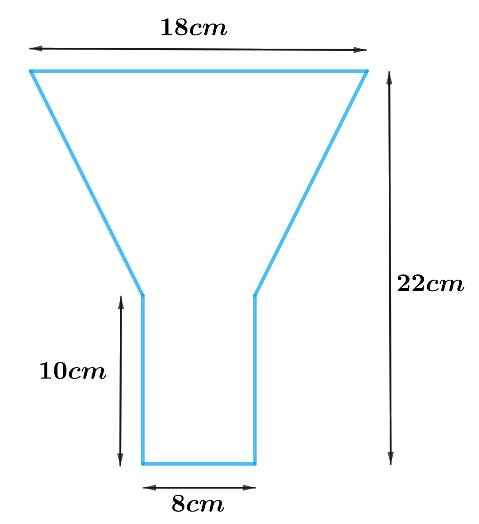### Solution

What is Known?

Length of the cylindrical part is $$10\,\rm{ cm}$$ and diameter is $$8 \,\rm{cm}$$. Diameter of top of the funnel is $$18\, \rm{cm}$$ and total height of funnel is $$22\,\rm{ cm.}$$

What is Unknown?

Area of tin sheets required to make the funnel

Reasoning: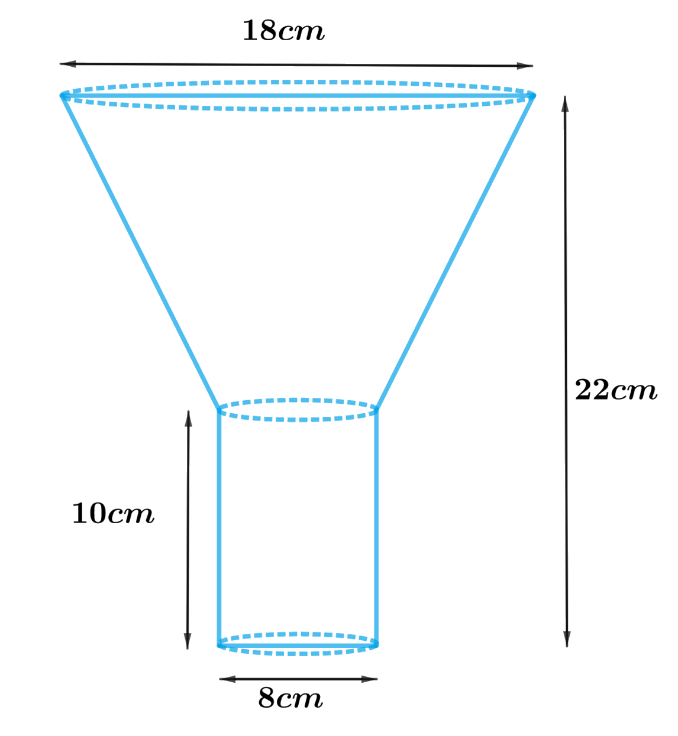Since the funnel is open at the top and the bottom. Hence, open at the junction of the frustum of cone and cylindrical part.

Therefore,

Area of tin sheet required to make the funnel $$=$$ CSA of frustum of cone $$+$$ CSA of the cylinder

We will find the CSA of the frustum by using formulae;

CSA of frustum of a cone $$= \pi \left( {{r_1} + {r_2}} \right)l$$

Slant height, $$l = \sqrt {{h^2} + {{\left( {{r_1} - {r_2}} \right)}^2}}$$

where $$r1, r2, h$$ and $$l$$ are the radii height and slant height of the frustum of the cone respectively.

CSA of the cylinder $$= 2\pi rh$$

where $$r$$ and $$h$$ are radius and height of the cylinder respectively.

Steps:

Height of the funnel, $$H = 22cm$$

Height of cylindrical part, $$h = 10cm$$

Height of frustum of cone, $${h_1} = 22cm - 10cm = 12cm$$

Radius of top part of frustum of cone, \begin{align}{r_1} = \frac{{18cm}}{2} = 9cm\end{align}

Radius of lower part of frustum of cone, \begin{align}{r_2} = \frac{{8cm}}{2} = 4cm\end{align}

Radius of cylindrical part, $${r_2} = 4cm$$

Slant height of frustum, \begin{align}l = \sqrt {{h_1}^2 + {{\left( {{r_1} - {r_2}} \right)}^2}}\end{align}

\begin{align}&= \sqrt {{{\left( {12cm} \right)}^2} + {{\left( {9cm - 4cm} \right)}^2}} \\&= \sqrt {144c{m^2} + 25c{m^2}} \\&= \sqrt {169c{m^2}} \\&= 13cm\end{align}

Area of tin sheet required to make the funnel $$=$$ CSA of frustum of cone $$+$$ CSA of the cylinder

\begin{align} & =\pi \left( {{r}_{1}}+{{r}_{2}} \right)l+2\pi {{r}_{2}}h \\ & =\pi \left[ \left( {{r}_{1}}+{{r}_{2}} \right)l+2{{r}_{2}}h \right] \\ & =\frac{22}{7}\left[ \begin{array} & \left( 9cm+4cm \right)\times 13cm+ \\ 2\times 4cm\times 10cm \\ \end{array} \right] \\ & =\frac{22}{7}\left[ 169c{{m}^{2}}+80c{{m}^{2}} \right] \\ & =\frac{22}{7}\times 249c{{m}^{2}} \\ & =\frac{5478}{7}c{{m}^{2}} \\ & =782\frac{4}{7}c{{m}^{2}} \\ \end{align}

## Chapter 13 Ex.13.5 Question 6

Derive the formula for the curved surface area and total surface area of the frustum of a cone, given to you in Section $$13.5$$, using the symbols as explained.

### Solution

What is Known?

A frustum of a cone with $$h$$ as height,$$l$$ as the slant height, $$r1$$ and $$r2$$ radii of the ends where $$r1 > r2$$

To prove:

(i) CSA of the frustum of the cone $$= \pi l\left( {{r_1} + {r_2}} \right)$$

(ii)TSA of the frustum of the cone \begin{align} = \pi l\left( {{r_1} + {r_2}} \right) + \pi r_1^2 + \pi r_2^2\end{align}

where $$r1, r2, h$$ and $$l$$ are the radii height and slant height of the frustum of the cone respectively.

Construction:

Extended side $$BC$$ and $$AD$$ of the frustum of cone to meet at $$O.$$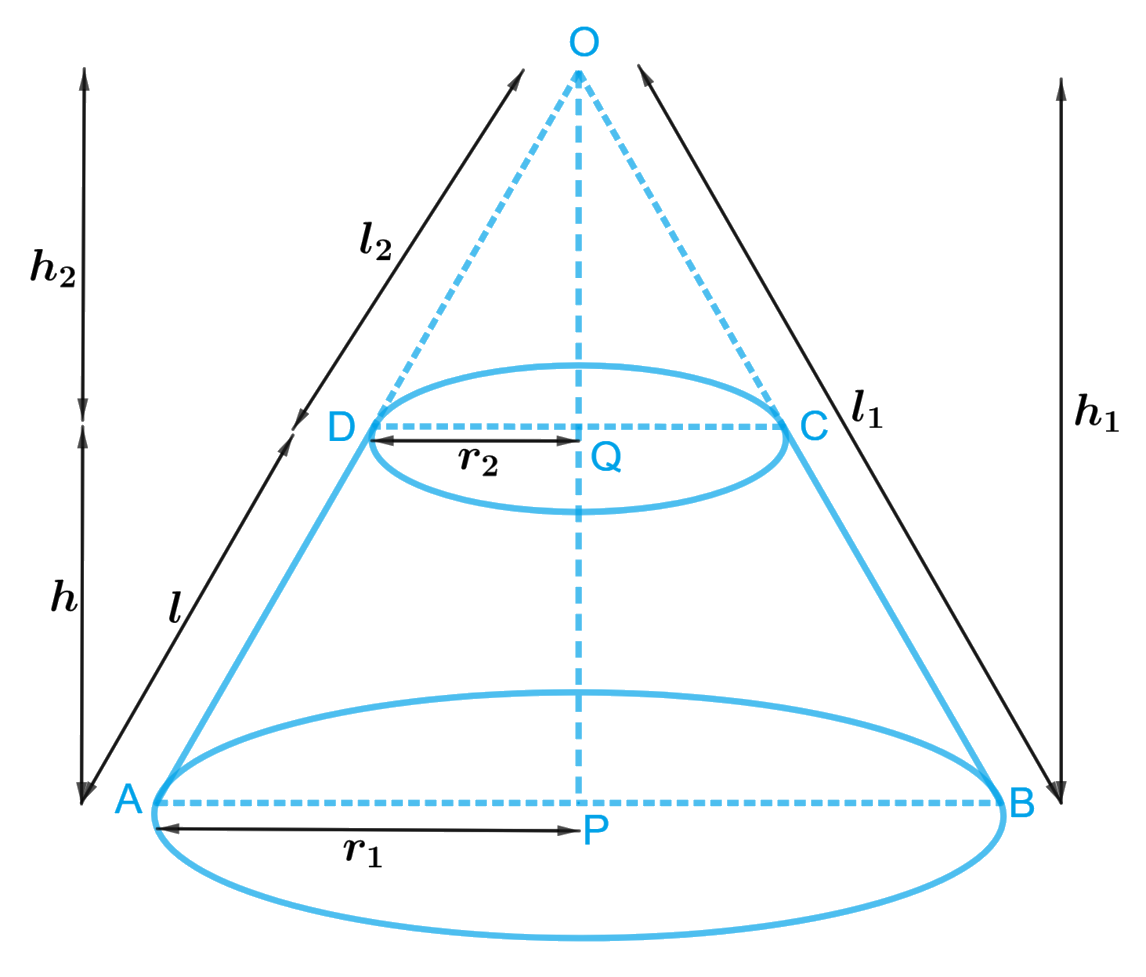Proof:

The frustum of a cone can be viewed as a difference of two right circular cones $$OAB$$ and $$OCD.$$

Let $$h1$$ and $$l1$$ be the height and slant height of cone $$OAB$$ and $$h2$$ and $$l2$$ be the height and slant height of cone $$OCD$$ respectively.

In $$\Delta APO$$ and $$\Delta DQO$$

$$\Delta APO = \Delta DQO = {90^\circ }$$ (Since both cones are right circular cones)

$$\angle APO = \angle DQO$$ (Common)

Therefore, $$\Delta APO \sim \Delta DQO$$ ( A.A criterion of similarity)

\begin{align}\frac{{AP}}{{DQ}} = \frac{{AO}}{{DO}} = \frac{{OP}}{{OQ}}\end{align} (Corresponding sides of similar triangles in proportion)

\begin{align} \Rightarrow \frac{{{r_1}}}{{{r_2}}} = \frac{{{l_1}}}{{{l_2}}} = \frac{{{h_1}}}{{{h_2}}}\end{align}

\begin{align} \Rightarrow \frac{{{r_1}}}{{{r_2}}} = \frac{{{l_1}}}{{{l_2}}} \\\text{or}\\\Rightarrow \frac{{{r_2}}}{{{r_1}}} = \frac{{{l_2}}}{{{l_1}}}\end{align}

Subtracting $$1$$ from both sides we get

\begin{align}\frac{{{r_1}}}{{{r_2}}} - 1 &= \frac{{{l_1}}}{{{l_2}}} - 1\\\frac{{{r_1} - {r_2}}}{{{r_2}}} &= \frac{{{l_1} - {l_2}}}{{{l_2}}}\\\frac{{{r_1} - {r_2}}}{{{r_2}}} &= \frac{l}{{{l_2}}}\\{l_2} &= \frac{{l{r_2}}}{{{r_1} - {r_2}}}{\rm\qquad{ (i)}}\end{align}

or

\begin{align} \Rightarrow \frac{{{r_2}}}{{{r_1}}} = \frac{{{l_2}}}{{{l_1}}}\end{align}

Subtracting $$1$$ from both sides we get

\begin{align}\frac{{{r_2}}}{{{r_1}}} - 1 &= \frac{{{l_2}}}{{{l_1}}} - 1\\\frac{{{r_2} - {r_1}}}{{{r_1}}} &= \frac{{{l_2} - {l_1}}}{{{l_1}}}\\\frac{{{r_1} - {r_2}}}{{{r_1}}} &= \frac{{{l_1} - {l_2}}}{{{l_1}}}\\\frac{{{r_1} - {r_2}}}{{{r_1}}} &= \frac{l}{{{l_1}}}\\{l_1} &= \frac{{l{r_1}}}{{{r_1} - {r_2}}}{\rm\qquad{ (ii)}}\end{align}

(i) CSA of frustum of cone $$=$$ CSA of cone $$OAB\, –$$ CSA of cone $$OCD$$

\begin{align} & =\pi {{r}_{1}}{{l}_{1}}-\pi {{r}_{2}}{{l}_{2}} \\& =\pi \left( {{r}_{1}}{{l}_{1}}-{{r}_{2}}{{l}_{2}} \right) \\& =\pi \left( \begin{array} & {{r}_{1}}\times \frac{l{{r}_{1}}}{{{r}_{1}}-{{r}_{2}}}-{{r}_{2}}\times \\ \frac{l{{r}_{2}}}{{{r}_{1}}-{{r}_{2}}} \\\end{array} \right) \\& \,\,\,\,\,\,\left[ \text{using}(\text{i})\text{and}(\text{ii}) \right] \\& =\pi \left( \frac{l{{r}_{1}}^{2}-l{{r}_{2}}^{2}}{{{r}_{1}}-{{r}_{2}}} \right) \\& =\pi \left( \frac{l\left( {{r}_{1}}^{2}-{{r}_{2}}^{2} \right)}{{{r}_{1}}-{{r}_{2}}} \right) \\& =\pi \left( \frac{l\left( {{r}_{1}}-{{r}_{2}} \right)\left( {{r}_{1}}+{{r}_{2}} \right)}{{{r}_{1}}-{{r}_{2}}} \right) \\& \,\,\,\,\left[ {{a}^{2}}-{{b}^{2}}=\left( a-b \right)\left( a+b \right) \right] \\& =\pi l\left( {{r}_{1}}+{{r}_{2}} \right) \\\end{align}

(ii)TSA of frustum of cone $$=$$ CSA of frustum $$+$$ Area of lower circular end $$+$$ Area of top circular end

\begin{align} = \pi l\left( {{r_1} + {r_2}} \right) + \pi r_1^2 + \pi r_2^2\end{align}

Therefore, CSA of the frustum of the cone \begin{align}= \pi l\left( {{r_1} + {r_2}} \right)\end{align}

TSA of the frustum of the cone\begin{align} = \pi l\left( {{r_1} + {r_2}} \right) + \pi r_1^2 + \pi r_2^2\end{align}

Hence Proved.

## Chapter 13 Ex.13.5 Question 7

Derive the formula for the volume of the frustum of a cone, given to you in Section $$13.5$$, using the symbols as explained.

### Solution

What is Known?

A frustum of a cone with $$h$$ as height, $$l$$ as the slant height, $$r1$$ and $$r2$$ radii of the ends where $$r1 > r2$$

To prove:

Volume of the frustum of a cone $$= \frac{1}{3}\pi h\left( {r_1^2 + r_2^2 + {r_1}{r_2}} \right)$$

Construction:

Extend side $$BC$$ and $$AD$$ of the frustum of cone to meet at $$O.$$Proof:

The frustum of a cone can be viewed as a difference of two right circular cones $$OAB$$ and $$OCD.$$

Let $$h1$$ and$$l1$$ be the height and slant height of cone $$OAB$$ and $$h2$$ and $$l2$$ be the height and slant height of cone $$OCD$$ respectively.

In $$\Delta APO$$ and $$\Delta DQO$$

$$\Delta APO = \Delta DQO = 90^\circ$$ (Since both cones are right circular cones)

$$\angle AOP = \angle DOQ$$ (Common)

Therefore,$$\Delta APO \sim \Delta DQO$$ ( A.A criterion of similarity)

\begin{align}\frac{{AP}}{{DQ}} = \frac{{AO}}{{DO}} = \frac{{OP}}{{OQ}}\end{align} (Corresponding sides of similar triangles are proportional)

\begin{align} \Rightarrow \frac{{{r_1}}}{{{r_2}}} = \frac{{{l_1}}}{{{l_2}}} = \frac{{{h_1}}}{{{h_2}}}\end{align}

\begin{align} \Rightarrow \frac{{{r_1}}}{{{r_2}}} = \frac{{{h_1}}}{{{h_2}}}\end{align} or \begin{align} \Rightarrow \frac{{{r_2}}}{{{r_1}}} = \frac{{{h_2}}}{{{h_1}}}\end{align}

Subtracting 1 from both sides

\begin{align}\frac{{{r_1}}}{{{r_2}}} - 1 &= \frac{{{h_1}}}{{{h_2}}} - 1\\\frac{{{r_1} - {r_2}}}{{{r_2}}} &= \frac{{{h_1} - {h_2}}}{{{h_2}}}\\\frac{{{r_1} - {r_2}}}{{{r_2}}} &= \frac{h}{{{h_2}}}\\{h_2} &= \frac{{h{r_2}}}{{{r_1} - {r_2}}}{\rm\qquad{ (i)}}\end{align}

or

\begin{align} \Rightarrow \frac{{{r_2}}}{{{r_1}}} = \frac{{{h_2}}}{{{h_1}}}\end{align}

Subtracting 1 from both sides we get

\begin{align}\frac{{{r_2}}}{{{r_1}}} - 1 &= \frac{{{h_2}}}{{{h_1}}} - 1\\\frac{{{r_2} - {r_1}}}{{{r_1}}} &= \frac{{{h_2} - {h_1}}}{{{h_1}}}\\\frac{{{r_1} - {r_2}}}{{{r_1}}} &= \frac{{{h_1} - {h_2}}}{{{h_1}}}\\\frac{{{r_1} - {r_2}}}{{{r_1}}} &= \frac{h}{{{h_1}}}\\{h_1} &= \frac{{h{r_1}}}{{{r_1} - {r_2}}}{\rm\qquad{ (ii)}}\end{align}

Volume of frustum of cone $$=$$ Volume of cone $$OAB$$ $$-$$ Volume of cone $$OCD$$

\begin{align}&= \frac{1}{3}\pi {r_1}^2{h_1} - \frac{1}{3}\pi {r_2}^2{h_2}\\&= \frac{1}{3}\pi \left( {{r_1}^2{h_1} - {r_2}^2{h_2}} \right)\\&= \frac{1}{3}\pi \left[{{r_1}^2 \times \frac{{h{r_1}}}{{{r_1} - {r_2}}} - {r_2}^2 \times \frac{{h{r_2}}}{{{r_1} - {r_2}}}} \right]\\&\qquad\left[ {{\rm{using (i) and (ii)}}} \right]{\rm{ }}\\&= \frac{1}{3}\pi \left[ {\frac{{h{r_1}^3}}{{{r_1} - {r_2}}} - \frac{{h{r_2}^3}}{{{r_1} - {r_2}}}} \right]\\&= \frac{1}{3}\pi h\left[{\frac{{{r_1}^3 - {r_2}^3}}{{{r_1} - {r_2}}}} \right]\\& = \frac{1}{3}\pi h\left[ {\frac{{\left( {{r_1} - {r_2}} \right]\left[ {{r_1}^2 + {r_1}^2{r_2}^2 + {r_2}^2}\right)}}{{{r_1} - {r_2}}}} \right]\\&\qquad\left[ {\left( {{a^3} - {b^3}} \right] = \left( {a - b} \right)\left( {{a^2} + ab + {b^2}} \right)} \right]\\&= \frac{1}{3}\pi h\left( {{r_1}^2 + {r_2}^2 + {r_1}^2{r_2}^2} \right)\end{align}

Volume of the frustum of a cone\begin{align} = \frac{1}{3}\pi h\left( {r_1^2 + r_2^2 + {r_1}{r_2}} \right)\end{align}

Hence Proved